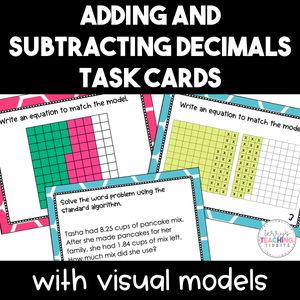• \$2.00
Unit price per
Shipping calculated at checkout.

Have your students practice adding and subtracting decimals with visual models and the standard algorithm with these sets of printable task cards.

Types of questions in each set of task cards:
• a visual model is provided and students create the matching equation
• an equation is provided and students create a visual model to match
• word problems are given and students will use the standard algorithm to solve.

Standard:  5.NBT.7